# Creating a Parameterized CurveThis task shows how to create a planar curve defined by two formulas.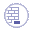You must have access to the Knowledge Advisor product.
Make sure the Relations and Parameters options are selected in the Tools > Options > Infrastructure > Part Infrastructure > Display tab.
Open a new .CATPart document.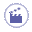1. Create two points using the following coordinates:

 Point.1: X=0, Y=0, and Z=22 Point.2: X=0, Y=0, and Z=75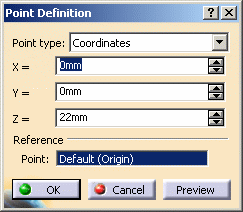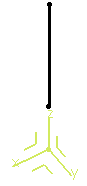2. Define line between these two points.

 It is created along the Z axis of the axis-system, using the Point-Point option.
3. From the Knowledge toolbar, click Law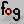.

 The Law Editor dialog box is displayed in which you name the law to be created, give it a description and a storage location.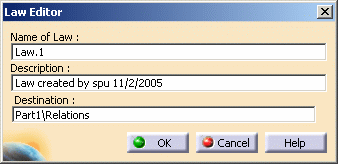4. Click OK.

 The Law Editor dialog box is updated. The right-hand part allows you to create the parameters to be used in the law. The left-hand part is the law edition box.
5. Create one real type parameter t and one length type parameter x, then enter the law below into the edition window: x=40mm+100mm*(t-0.5)**2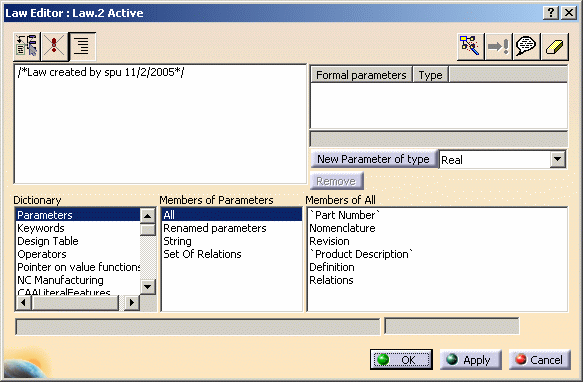6. Click OK to create the law.

7. Create a second law using one real type parameter t and one length type parameter y, then entering the following law into the edition window: y=40mm+200mm*(t-0.5)*(0.25-t)*(0.75-t)

8. Create a parallel curve, using the Line.1 created in step 2 as the reference Curve to be offset, and the ZX plane of the axis system as the Support on which the reference curve lies.

9. Click Law... and select Law.1 from the specification tree.

10. Click OK.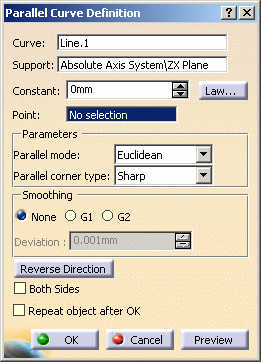A curve parallel to the selected one is created, taking the law into account, i.e. it is defined by the equation entered as a law using the Knowledge Advisor.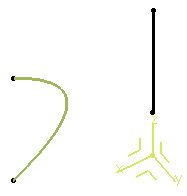11. Create a second parallel curve, using Line.1 as the reference Curve and the YZ plane as the Support.

12. Click Law... and select Law.2.

13. Click OK to create the parallel curve with a variable law.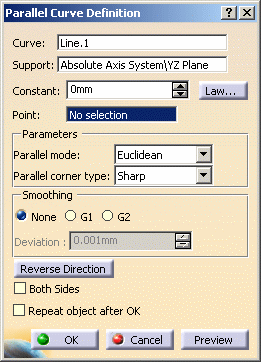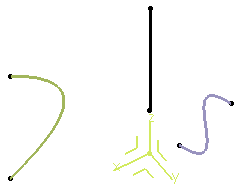14. Use the Combine command to create a curve resulting from the intersection of the extrusion of the two parallel curves.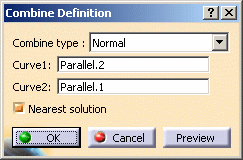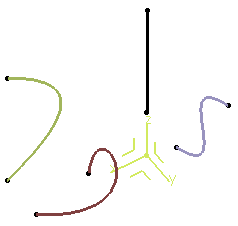15. Use the Project command to project the combined curve onto the xy plane:

 Choose the combined curve as the element to be projected, the xy plane as the Support, and the Z axis as the Direction.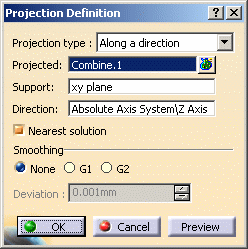Here is the parameterized curve (in blue) obtained by two formulas: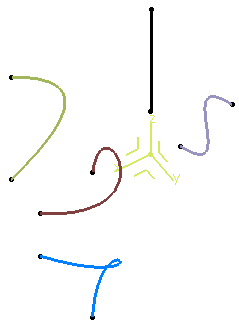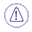It is not advised to create closed parameterized curves.